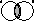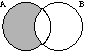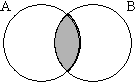Previous Page Up One Level

Lecture Slides available: PDF PowerPoint

# Relational Algebra - Example

## Contents

Consider the following SQL to find which departments have had employees on the `Further Accounting' course.

``` SELECT DISTINCT dname
FROM  department, course, empcourse, employee
WHERE cname = `Further Accounting'
AND course.courseno = empcourse.courseno
AND empcourse.empno = employee.empno
AND employee.depno = department.depno;
```

The equivalent relational algebra is

``` PROJECTdname (department JOINdepno = depno (
PROJECTdepno (employee JOINempno = empno (
PROJECTempno (empcourse JOINcourseno = courseno (
PROJECTcourseno (SELECTcname = `Further Accounting' course)
))
))
))
```

## Symbolic Notation

From the example, one can see that for complicated cases a large amount of the answer is formed from operator names, such as PROJECT and JOIN. It is therefore commonplace to use symbolic notation to represent the operators.

• SELECT ->σ (sigma)
• PROJECT -> π(pi)
• PRODUCT -> ×(times)
• JOIN -> |×| (bow-tie)
• UNION -> ∪ (cup)
• INTERSECTION -> ∩(cap)
• DIFFERENCE -> - (minus)
• RENAME ->ρ (rho)

## Usage

The symbolic operators are used as with the verbal ones. So, to find all employees in department 1:

``` SELECTdepno = 1(employee)
becomes σdepno = 1(employee)
```

Conditions can be combined together using ^ (AND) and v (OR). For example, all employees in department 1 called `Smith':

``` SELECTdepno = 1 ^ surname = `Smith'(employee)
becomes σdepno = 1 ^ surname = `Smith'(employee)
```

The use of the symbolic notation can lend itself to brevity. Even better, when the JOIN is a natural join, the JOIN condition may be omitted from |x|. The earlier example resulted in:

``` PROJECTdname (department JOINdepno = depno (
PROJECTdepno (employee JOINempno = empno (
PROJECTempno (empcourse JOINcourseno = courseno (
PROJECTcourseno (SELECTcname = `Further Accounting' course)))))))
```

becomes

``` πdname (department |×| (
πdepno (employee |×| (
πempno (empcourse |×| (
πcourseno (σcname = `Further Accounting' course) ))))))

```

## Rename Operator

The rename operator returns an existing relation under a new name. ρA(B) is the relation B with its name changed to A. For example, find the employees in the same Department as employee 3.

``` ρemp2.surname,emp2.forenames (
σemployee.empno = 3 ^ employee.depno = emp2.depno (
employee × (ρemp2employee)
)
)
```

## Derivable Operators

• Fundamental operators:σ, π, ×, ∪, -, ρ
• Derivable operators: |×|,∩
 A ∩ B ⇔ A - (A - B)## Equivalence

```A|×|cB ⇔ πa1,a2,...aN(σc(A × B))
```
• where c is the join condition (eg A.a1 = B.a1),
• and a1,a2,...aN are all the attributes of A and B without repetition.

c is called the join-condition, and is usually the comparison of primary and foreign key. Where there are N tables, there are usually N-1 join-conditions. In the case of a natural join, the conditions can be missed out, but otherwise missing out conditions results in a cartesian product (a common mistake to make).

## Equivalences

The same relational algebraic expression can be written in many different ways. The order in which tuples appear in relations is never significant.

• A ×B ⇔ B × A
• A ∩ B ⇔ B ∩ A
• A ∪B ⇔ B ∪ A
• (A - B) is not the same as (B - A)
• σc1c2(A)) ⇔ σc2c1(A)) ⇔ σc1 ^ c2(A)
• πa1(A) ⇔ πa1a1,etc(A))
where etc represents any other attributes of A.
• many other equivalences exist.

While equivalent expressions always give the same result, some may be much easier to evaluate that others.

When any query is submitted to the DBMS, its query optimiser tries to find the most efficient equivalent expression before evaluating it.

## Comparing RA and SQL

• Relational algebra:
• is closed (the result of every expression is a relation)
• has a rigorous foundation
• has simple semantics
• is used for reasoning, query optimisation, etc.
• SQL:
• is a superset of relational algebra
• has convenient formatting features, etc.
• provides aggregate functions
• has complicated semantics
• is an end-user language.

## Comparing RA and SQL

Any relational language as powerful as relational algebra is called relationally complete.

A relationally complete language can perform all basic, meaningful operations on relations.

Since SQL is a superset of relational algebra, it is also relationally complete.

 Previous Page Up One Level